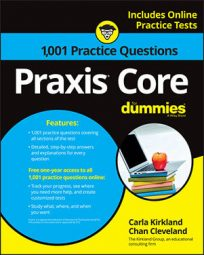##### Praxis Core: 1,001 Practice Questions For DummiesRatios are often used to compare numbers—sports scores, for example—so don't be surprised if you encounter a ratio problem on the Praxis Core exam.

In the following practice questions, you start by finding the ratio of a golfer's score from one day to another; then you need to find the ratio of football players enrolled in two different classes.

## Practice questions

1. In a game of golf, Johnny scored 78. The next day, Johnny played another game of golf and scored 72. What is the ratio of Johnny's golf score on the first day to his score on the second day, in simplest form?

2. In a classroom, 8 of the 30 students are football players. In another classroom, 3 of the 10 students are football players. What is the sum of the ratios of football players to students who do not play football for the two classrooms?

1. The correct answer is Choice (A). The ratio of Johnny's golf score on the first day to his score on the second day is 78 to 72, or

To write that fraction in simplest form, divide the numerator and denominator by their greatest common factor, which is 6:

2. The correct answer is Choice (E). Because the number of football players in the first classroom is 8 and there are 30 students in the classroom, the ratio of students who play football to students who do not is

which can be reduced to

In the second classroom, 3 of the 10 students play football, so 7 do not. The ratio of students who play football to students who do not in that classroom is

You can add the two ratios to get their sum: